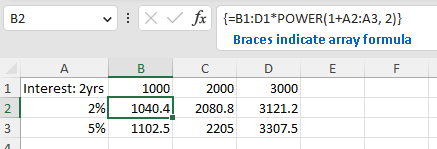# Formulas

## Feature overview

Cell of a workbook can have formulas, i.e. a recipe to calculate the output value from other cell values, constants, operations and functions.

Excel supports four types of formulas:

• Normal - Formula calculates a single value and the result of the calculation is set as a value of the cell.

• Array - A range of cells are calculated at once, using a single formula. Array formula can be set even on a single cell, which results in a 1x1 array formula.

• Data table - Used for what-if analysis, uses a formula along with a set of values to calculates a range of values (e.g. calculate monthly payment based on the mortgage rate and amount financed).

• Shared - this is basically a memory optimization, where many cells reference the same formula.

## Available API

### Array formulas

#### Set an array formula

Select an area of cells to set the array formula and then set the formula through IXLRangeBase.FormulaArrayA1 setter.

```using var wb = new XLWorkbook();
ws.Cell("A1").Value = "Interest: 2yrs";
ws.Cell("B1").Value = 1000;
ws.Cell("C1").Value = 2000;
ws.Cell("D1").Value = 3000;
ws.Cell("A2").SetValue(0.02)
.Style.NumberFormat.SetNumberFormatId((int)XLPredefinedFormat.Number.PercentInteger);
ws.Cell("A3").SetValue(0.05)
.Style.NumberFormat.SetNumberFormatId((int)XLPredefinedFormat.Number.PercentInteger);

ws.Range("B2:D3").FormulaArrayA1 = "B1:D1*POWER(1+A2:A3, 2)";

wb.SaveAs("array-formula-create.xlsx");
```When a cell is a part of an array formula,

• its `IXLCell.FormulaA1` getter will return the array formula (no braces).

• the `IXLCell.HasArrayFormula` getter return `true`

• the `IXLCell.FormulaReference` getter return the area of the array formula.

It’s not possible to create an array formula that would intersect with a merged range, table or partially overlaps another array formula. Doing so will throw `InvalidOperationException`. If new array formula completely covers existing array formula, there isn’t an exception.

#### Clear array formula

In order to clear an array formula, set a range covering the formula to a value. Any value will do, though it will be `Blank.Value` in most cases. The range can be determined through the `IXLCell.FormulaReference` property or explicitely set.

```using var wb = new XLWorkbook();